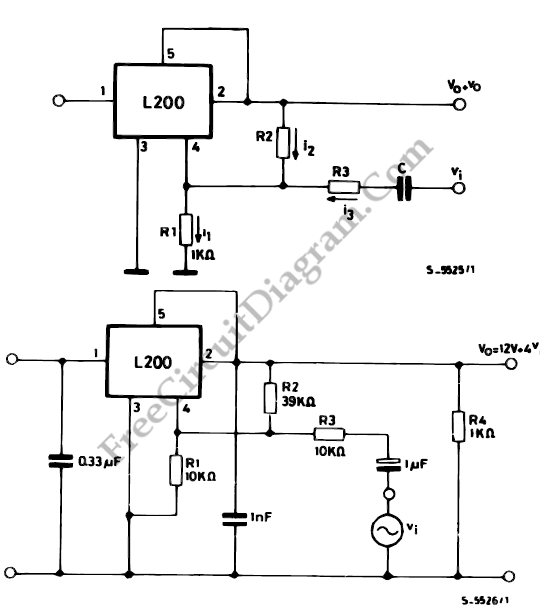# Power Amplitude Modulator

We can use L200 to send a signal onto a supply line. We can define Vo as Vo = Vref (1+ R2/R1)  since the input signal Vi is DC decoupled.The gain of amplified signal is:

Gv= -R2/R1

This amplified signal is added to this component. We must impose i1 = i2+i3 (1) by ignoring the current entering pin 4.

Equation (1) becomes

i2= -i3 with i3 = Vi/R3 (for Xc<<R3)

Vo=R2 i2= -(Vi/R3).R2

since the voltage between pin 4 and ground remains fixed (Vref) as long the device is not in saturation, i1=0.

An application is shown in circuit diagram below. R1 should be replaced by a potentiometer if the DC level is to be varied but not the AC gain. [Source: STMicroelectronics Application Note]